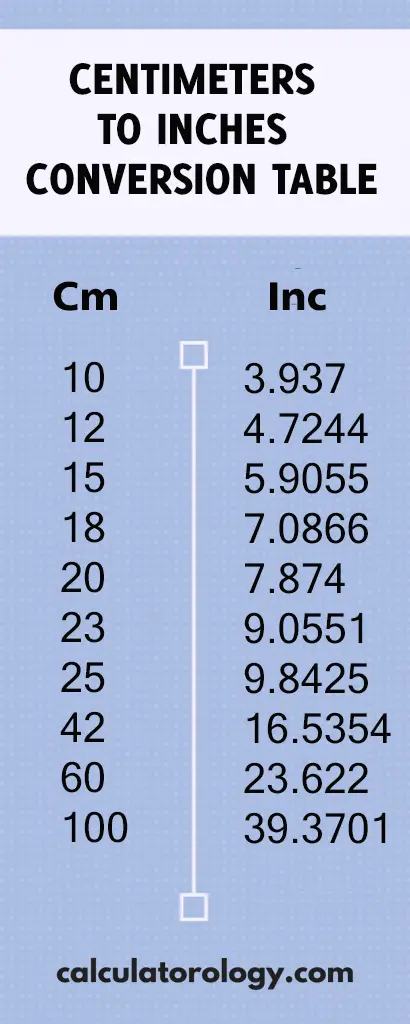## 7.0866141732 inches

### 18cm in inches

The conversion factor of centimeters (cm) to inches (in) is 0.3937007874. If you want to determine the number of inches in centimeters, you simply multiply the value centimeters by 0.3937007874. The conversion calculator can also be used to perform more conversions as it uses the same formula to give quick and accurate results. The first procedure is to enter the value in centimeters (18) in the blank text field and then click on the ‘Convert’ button to initiate the conversion.

To determine how many inches are in 18cm, it is important to determine the number of inches that make up one centimeter.

#### How many inches in one centimeter?

1 cm = (1/2.54)” = 0.3937007874

This means that there are 0.3937007874 inches in one centimeter.

D (in) = D (cm) / 2.54

The distance in inches is determined by dividing the value in centimeters by 2.54.## 18cm in Inches Conversion

 cm inches

#### How to convert 18cm to inches

Since 1 centimeter is equivalent to 0.3937007874 inches, how many inches are there in 18 centimeters?

If 1 cm = 0.3937007874 inches,

18cm =? Inches

Calculation:     18cm / 2.54

= 7.0866141732"

Inches fraction result:  7 3/32

Feet+inches result:       0ft 7.0866141732

This implies that there are 7.0866141732inches in 18 centimeters.

The conversion calculator can be used to perform other conversions using different values. The centimeter to inches conversion calculator can only execute a single conversion at a time. The ‘Reset’ button is used to erase all information of the previous calculations to create a new platform for more conversions.18 cm to inches can also be determined using the Centimeter to Inches conversion table. It gives the conversion results of centimeters to inches based on a range of 0.01cm to 100cm.

##### Definitions

A centimeter is a length unit in the metric system abbreviated as "cm". One centimeter is equal to 0.3937007874 inches.

An inch is a US customary and imperial measurement system that is used widely and is abbreviated as (in) or using the symbol (“) - double prime.